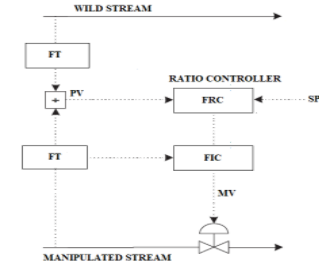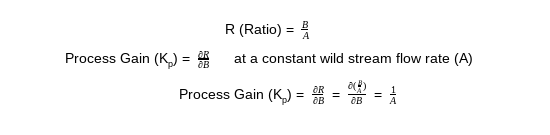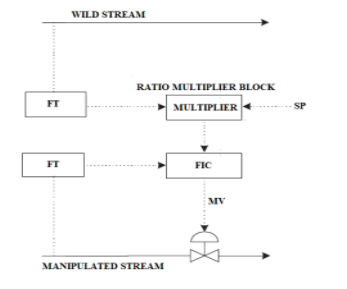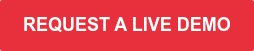Ratio control is an essential element in the toolbox of control engineers to control various industrial processes. The objective is to maintain the ratio of two flows at a specified value. The desired ratio can be either manually entered by an operator or automatically set by another controller. At least one of the flows needs to be controlled by a PID controller (or at least a valve should be available to control the flow). The ratio can be calculated based on the flow measurements or based on the setpoints of the flow controller(s).  The ratio controller can be configured in different ways in the DCS. The different approaches and their pro’s and con’s are described in this blog.

## Using PV or SP for the ratio calculation?

Most ratio controllers are calculating the ratio based on the Process values (PV) of the transmitters. This arrangement is simple but could pose a risk of oscillation if the dynamics of one of the flow controllers are relatively slow and frequent disturbances affect the flow.

Consider a situation in which the flow controller is relatively slow, and the measured flow deviates from its setpoint.  Based on the measured flow, the calculated ratio will deviate from its target since the flow deviates from setpoint. This will cause the ratio controller to take action and adjust the setpoint of the flow controller.  However, that flow controller is still in the process of correcting the flow, bringing it back to its setpoint. In other words: the ratio controller is correcting for a disturbance that the flow controller should handle. This could lead to oscillations. In these cases, it is advised to use the flow controller setpoint(s) for the ratio calculation.  This will make sure that the ratio controller lets the flow controller “do its job” to keep the flow on setpoint.

In case there are process-reasons for using the PV’s of the flows instead of the SP’s, the ratio controller should be tuned a bit slower than the slowest flow controller, to prevent the oscillations described above.## Using a PID block or a multiplier block?

Some DCS’s have a standard ratio block available in the DCS. In all other cases, a ratio controller can be configured in the DCS using a PID block or a multiplier block.  Both have their pro’s and con’s.

Figure A below shows the setup with a PID block. In this example, one of the two flows is uncontrolled (the “wild stream”). The other stream is controlled with a flow controller. The ratio of the two streams is calculated by a calculation block, taking the PV’s of the flows. This calculated ratio is fed to a PID controller as the PV. The PID controller's output goes to the setpoint of the flow controller.Figure A

One of the advantages of this control scheme is that a calculated ratio value is available in the DCS, which can be used for alarming etc. Another advantage is that the use of a PID block provides the operator with a standard faceplate, enables easy cascading to another controller (e.g. temperature control in a reactor), and enables easy integration with an Advanced Process Control (APC) application.

A potential disadvantage is that the process gain is nonlinear, because of the fact that the ratio is the quotient of two flows. For further clarification, assume that A is an uncontrolled “wild stream”, and B is a controlled flow rate:The process gain is inversely related to the wild stream flow rate (A) from the above equation.

If the range in which the ratio is to be controlled is relatively narrow compared to the full range of the ratio based on the ranges of the flow instruments, then the nonlinearity is not a problem. If the ratio is to be controlled over a wide range, and the nonlinearity is expected to be an issue, an alternative method can be used.

In Figure B below, the “wild stream” flow measurement is sent to a multiplier block where it is multiplied by the desired ratio and sent as the setpoint to the flow controller. The main advantage is that there is no non-linearity in this control scheme. Also, there is no need to configure and tune a PID block for ratio control. The disadvantage is that in this scheme the actual ratio is not available as a calculated variable, so to show this ratio to the operator, one would need to build a separate calculation block.  In addition, a manual entry point would have to be created in which the operator can enter the desired ratio.  Cascading this scheme to other controllers may not be trivial either.Figure B

## Ratio control is powerful!

Ratio control is a great tool to bring stability and automation to your plant.  With a correct ratio control configuration, you can gain benefits like:

• Tight ratio control
• More process stability
• Reduced alarms
• Reduced operator interventions

Do you want to learn more about base layer configuration or PID tuning software, how improved PID tuning stabilizes your plant and reduces alarms and operator interventions? Request a 30-minute live demo and gain insights into what you can achieve with INCATools PID Tuning. It's all you need to get your PID tuning the first time right.Topics: PID tuning